## General Question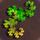# Logic Problem: How to separate the real and counterfeit coins in the fewest number of turns?

Asked by PhiNotPi (12677) February 28th, 2012

There are 16 coins. An unknown number K of them are counterfeit and need to be separated. All of the coins look identical and feel identical, except that they are lettered A-P. Luckily, there is a machine that will accept any number of coins at once and return a “yes” is there is at least one counterfeit coin in that sample, and “no” otherwise. (all of the coins are returned)

The goal is to find a strategy that finds ALL counterfeit coins in the fewest number of turns (each use of the machine is one turn).

One strategy is to use the machine 16 times to test each coin one by one; however, if you test a large number of coins and get a “no” response, then you know that all of the coins are real and can save some turns.

Observing members: 0Composing members: 0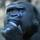This is very likely wrong, but my gut says to divide the stack into 4 piles of 4. Test each batch, and then further subdivide batches that come out as positive by 2 and then finally individually. I could very well be wrong about this, but the efficiency would depend on the value of K. As K increases, all solutions would approach the worst-case scenario. Actually my system would be much more inefficient than doing 16 individual tests if all coins were counterfeit. Interesting puzzle. Do you know the answer, or are you just trying to solve the dilemma?

gorillapaws (27309)“Great Answer” (1) Flag as…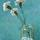Use Algebra.

whitecarnations (1635)“Great Answer” (0) Flag as…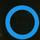Never mind, I misread the question and thought it was one counterfeit coin, I would have to rework it to figure out how many turns I need.

amujinx (5165)“Great Answer” (0) Flag as…So I wrote a python program to simulate my strategy for each value of K 10,000 times and here were my results:

K = 1 , Average = 6.5064
K = 2 , Average = 8.7596
K = 3 , Average = 10.7244
K = 4 , Average = 12.4352
K = 5 , Average = 13.9766
K = 6 , Average = 15.2918
K = 7 , Average = 16.4134
K = 8 , Average = 17.3508
K = 9 , Average = 18.1082
K = 10 , Average = 18.704
K = 11 , Average = 19.1872
K = 12 , Average = 19.5428
K = 13 , Average = 19.7954
K = 14 , Average = 19.934
K = 15 , Average = 20.0

If we assume that it’s equally likely that K could be any value between 1 and 15, my approach yielded an average of 15.781986666666665 steps to solve the problem which is negligibly better than testing each one. It appears that as long as K < 7, my approach is better than testing them individually. If K >= 7 then you’re better off testing them all individually.

There is probably a better approach. I should also note that it’s possible I made a logic error in my code which would mean my results could be significantly off.

gorillapaws (27309)“Great Answer” (1) Flag as…I ran the simulation again, this time dividing the stack into 8 groups of 2 and testing each pair first before testing individuals. The results were much better:

K = 1 , Average = 8.2516
K = 2 , Average = 8.4882
K = 3 , Average = 8.6952
K = 4 , Average = 8.893
K = 5 , Average = 9.0816
K = 6 , Average = 9.2386
K = 7 , Average = 9.4144
K = 8 , Average = 9.5442
K = 9 , Average = 9.6434
K = 10 , Average = 9.759
K = 11 , Average = 9.827
K = 12 , Average = 9.8974
K = 13 , Average = 9.9532
K = 14 , Average = 9.9858
K = 15 , Average = 10.0

Again, assuming an equal probability that K would be any value between 1 and 15 (inclusive), the average was a much sexier 9.378173333333335.

edit: I don’t think these numbers can be correct. I’m going to re-examine my code.

gorillapaws (27309)“Great Answer” (1) Flag as…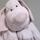@gorillapaws
I don’t understand your average at K=15 (K could also be 16, BTW.)

If fifteen are counterfeit then the following will happen:

1) test first pair result: yes
2) test #1 of first pair, result: yes
3) test #2 of first pair, result: yes
4) test second pair result: yes
5) test #1 of second pair, result: yes
6) test #2 of second pair, result: yes
7) test third pair result: yes
8) test #1 of third pair, result: yes
9) test #2 of third pair, result: yes
10) test 4th pair result: yes
11) test #1 of 4th pair, result: yes
12) test #2 of 4th pair, result: yes

At one moment (K=15) you will get a ‘no’ but that doesn’t help you in not having to test all other coins. Your average at K=15 will be 24 tests, not 9.something.

(I think you tested a pair only twice, but that doesn’t suffice, because you don’t know how many are counterfeit, so after having tested a pair, you’d still have to check each member of that pair.)

whitenoise (14126)“Great Answer” (1) Flag as…I’d do the following:

1) test the whole batch. If no, then done.
2) if yes divide in two batches and repeat process for each batch.

Always set aside batches that have no as answer as totally clean.

Don’t know if that makes sense, but intuitively that would be my choice. Would take more time than I have to think of mathematical foundation.

whitenoise (14126)“Great Answer” (2) Flag as…@whitenoise The reason that do not I think that testing the whole batch as the first step is the best is that there is only one case (a 1/17 chance) that it will say “no” and you will gain much useful information.

PhiNotPi (12677)“Great Answer” (0) Flag as…There are 2^16 possibilities. At each turn there are two possible results, so 16 turns collectively have 2^16 possible outcomes.. You can’t do better than 16 turns to distinguish all the possibilities.

LostInParadise (28939)“Great Answer” (0) Flag as…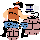I think that overall you are not going to be able to do better than a straight binary search.

Divide the stack in two equal piles. Run one pile through. If it comes up with a yes, divide again into two piles. Repeat on all remaining piles.

YoBob (12833)“Great Answer” (0) Flag as…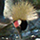Does it make sense to vary the trial size according to the earliest results?

6rant6 (13692)“Great Answer” (0) Flag as…@YoBob as K becomes larger, a binary search becomes MUCH worse than checking them all.

@whitenoise That’s essentially what the intent of my code is doing, although I’m sure I made a mistake with the last group. I haven’t had a chance to sort it out just yet though.

gorillapaws (27309)“Great Answer” (0) Flag as…Hmm… on second thought you are right @gorillapaws, as K increases to a values greater than half the number of total coins the binary search totally falls apart.

YoBob (12833)“Great Answer” (0) Flag as…I wonder if information theory could be used for this problem. Instead of assuming that the probability for each value of K is the same, assume the probability of a coin being counterfeit is p, with the coin probabilities being independent. Unfortunately, I do not know much information theory. The information contained in a coin being counterfeit is -p(lg(p)) and of not being counterfeit is -(1-p)(lg(1-p)). The idea would be, for a given p, to determine the average information returned for given weighing strategy.

LostInParadise (28939)“Great Answer” (0) Flag as…Another idea occurred to me. Suppose the coins have some unknown probability p of being counterfeit. Perform some number n of tests of individual coins, turning up k counterfeits. Estimate p as k/n and choose a strategy accordingly. One simple approach would be that if p exceeds some threshold then continue looking at one coin at a time. Otherwise, switch to binary tests.

LostInParadise (28939)“Great Answer” (1) Flag as…I think @LostInParadise has it right with the unknown probability. The way the problem is stated it is possible for all coins to be counterfeit, in which case the best you can achieve is checking them all individually. If, however, you know the number of counterfeits, or even have a probability of number of counterfeits then you can optimize the strategy accordingly.

YoBob (12833)“Great Answer” (0) Flag as…Here’s something neat- If we consider the total number of counterfeit coins to have a uniform distribution, then you can actually use the results from some tests to adjust the expected number of counterfeit coins in the sample that you did not test.

Here’s an example with 2 coins instead of 16.

There are four unique ways (because they are ordered by letter) that the coins can be real or counterfeit:
R R
R C
C R
C C
If we look at this, there is a 2/4 chance that there is one fake coin, but this is not a uniform distribution. The correct way is below, with combinations repeated to show which ones are more likely.
R R
R R
R C
C R
C C
C C
Now, the totals of zero, one, and two counterfeits all occur equally often.

Here’s the neat part: If you test Coin A and find out that it is real, then there is a ⅔ chance that the Coin B is also real, based on the above chart of the combinations and their likelihoods.

However, I am not quite sure if this is the correct way to interpret this problem. I am actually fairly sure that it is supposed to be interpreted as each individual coin has a set probability of being real or counterfeit.

PhiNotPi (12677)“Great Answer” (0) Flag as…or